Next:9.8 Lower Bound on Complexity for Sorting MethodsUp:9.7 Order StatisticsPrevious:9.7.2 Algorithm 2

## 9.7.3 Algorithm 3

Worst case linear time algorithm due to

Blum, Floyd, Pratt, Rivest, Tarzan.

REF.
Manuel Blum, Robert W. Floyd, Vaughan Pratt, Ronald L Rivest, and Robert E. Tarjan. Time bounds for selection. Journal of Computer and System Sciences, Volume 7, Number 4, pp. 448-461, 1973.
• This is also a variation of quicksort and the main idea is to find a good pivot.
• Assume that all the elements are distinct. The algorithm works otherwise too.
1.
Divide the n elements into groups of 5 leaving aside between 0 and 4 elements that cannot be placed in a group.

Sort each group of 5 elements by any algorithm and take the middle element from each group. This yields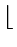n/5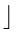medians.

2.
Use the SELECT algorithm to find the median of thesen/5elements. Choose this median as the pivot.
• Note that this pivot is in position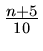• Also, the pivot exceeds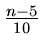of the middle elements and each of these middle elements exceeds two elements. Thus, the pivot exceeds at least
3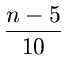elements.
Also, by a similar argument, the pivot is less than at least

3elements.
• If n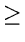75,
33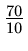= 21 >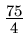In other words,

n753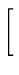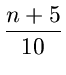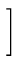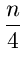This would mean that, if n75, the pivot is greater than at least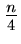elements and less than at leastelements. Consequently, when we partition an array with this pivot, the kth element is isolated to within a range of at most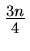of the elements.

Implementation

keytype select (int i, j, k);

/* returns key of the kth largest element among A[i ...  A[j]*/

{

if((j - i) < 75)

find the kth largest by some simple algorithm

else {

for(m = 0;m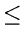(j - i - 4)/5;m + +)

{

find the third element among A[i + 5 * m ...  A[i + 5 * m + 4]

and swap it with A[i + m];

pivot = select (i,(j - i - 4)/5,(j - i - 4)/10)

m = partition (i, j, pivot);

if(km - i)

return (select (i, m - 1, k))

else

return (select (m, j,(k - (m - i)))

}

}

}

The worst case complexity of the above algorithm can be described by

 T(n)C1  if n75C2n + T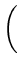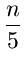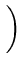+ T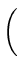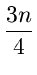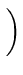if n > 75

Let us guess the solution T(n) = Cn for n > 75. For n75, assumeT(mCm for m < n. Then

 T(n)C2n +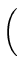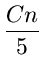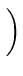+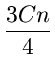C2n +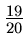Cn

Thus T(n) is O(n).

• Instead of groups of 5, let us say we choose groups of 7. It is easy to show that the worst case complexity is again O(n). In general, any size of the groups that ensures the sum of the two arguments of T(.) to be less than nwill yield O(n) worst case complexity.Next:9.8 Lower Bound on Complexity for Sorting MethodsUp:9.7 Order StatisticsPrevious:9.7.2 Algorithm 2
eEL,CSA_Dept,IISc,Bangalore